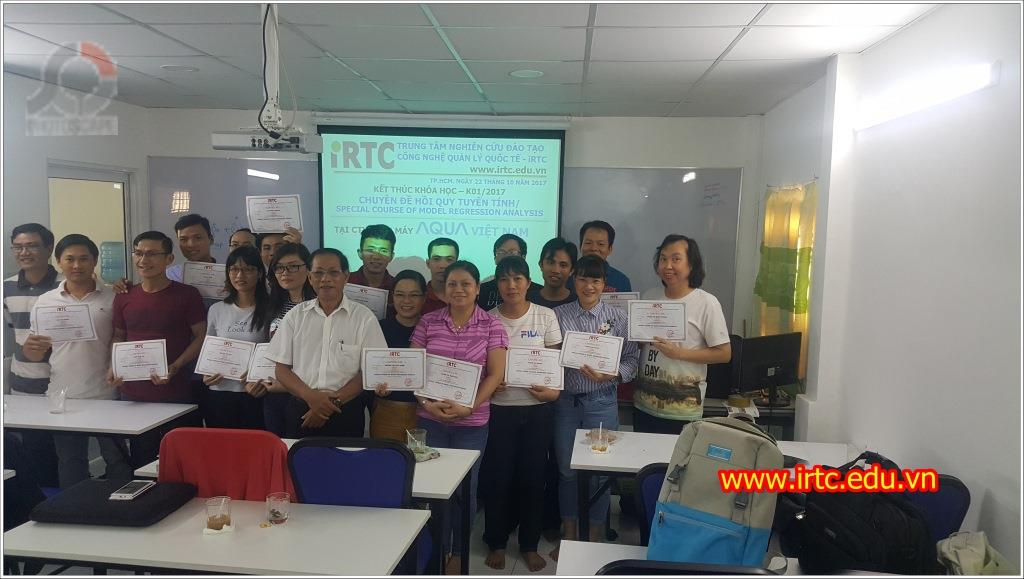### KHÓA CHUYÊN ĐỀ HỒI QUY TUYẾN TÍNH - SPECIAL COURSE OF MODEL REGRESSION ANALYSIS

(Khai giảng ngày 08/12/2019, học chủ nhật hàng tuần)

Thời gian học: Chủ nhật hàng tuần
(Sáng 08h30 - 11h30; Chiều 13h00 - 16h00)SPECIAL COURSE OF MODEL REGRESSION ANALYSIS

Mô hình hồi qui được sử dụng trong giai đoạn phân tích (ANALYSE) phân tích các dữ liệu trong quá trình áp dụng Six Sigma ( Define, Measure, Analyse, Improve , Control) nhằm tiên đoán và đưa ra các quyết định tốt nhất về quản trị dựa trên các biến tiên lượng ( Resourcse – nguồn lực) đang thực hiện.
Nhà quản trị xác định các yêu cầu liên quan đến dịch vụ và sản phẩm Công ty cung cấp KPOV – Key Process Output Variable và xác định các ảnh hưởng của nguồn lực khi thực hiện quá trình KPIV – Key Process Input Variable để tìm hiểu mối quan hệ của các biến liên quan nhằm chọn một mô hình tối ưu trước khi ra quyết định quản trị.
Khóa học chuyên đề về Phân tích Mô hình hồi quy Học viên sẽ được thực tập các tình huống trên 02 phần mềm Excel và Minitab

Nội dung chương trình:

 Date Time Topic Contents Day 1 8h30 - 11h30 Lesson 1: Simple Linear Regression Simple Linear Regression introduce Best Fit Line The Simple Linear Regression Model The Common Error Variance (Pearson) Correlation Coefficient r R-squared Cautions Hypothesis Test for the Population Correlation Coefficient Inference for the Population Intercept and Slope 13h00 - 16h00 Lesson 2: SLR Model Evaluation Lesson 3: SLR Estimation & Prediction The Analysis of Variance (ANOVA) table and the F-test The Lack of Fit F-test Confidence Interval for the Mean Response Prediction Interval for a New Response Further Example Day 2 8h30 - 11h30 Lesson 4: Multiple Linear Regression The Multiple Linear Regression Model A Matrix Formulation of the Multiple Regression Model Further Examples 13h00 - 16h00 Lesson 5: MLR Model Evaluation MLR Estimation, Prediction & Model Assumptions Three Types of Hypotheses The General Linear F-Test The Hypothesis Tests for the Slopes Lack of Fit Testing in the Multiple Regression Setting Prediction Interval for a New Response MLR Model Assumptions Day 3 8h30 - 11h30 Lesson 6: Categorical Predictors The Basics Two Separate Advantages Additive Effects and Interaction Effects Leaving an Important Interaction Out of a Model 13h00 - 16h00 Lesson 7: Multicollinearity Multicollinearity introduction Detecting Multicollinearity Using Variance Inflation Factors Reducing Data-based Multicollinearity Reducing Structural Multicollinearity Day 4 8h30 - 11h30 Lesson 8: Logistic and Poisson Regression Logistic Regression Polytomous Logistic Regression Poisson Regression 13h00 - 16h00 Lesson 9: Nonlinear and Exponential Regression Generalized Linear Models Nonlinear Regression Exponential Regression Example

Lecturer : LEAN SIX SIGMA MASTER BLACK BELT. MBA. LA. Mr.Kieu Van Ton
Số buổi học : 08 buổi/khóa (4 ngày)
Học phí : 4.800.000 VNĐ/khóa
Ưu đãi : Giảm còn 4.500.000 VNĐ/khóa dành cho học viên đã học tại iRTC hoặc nhóm ≥ 03 người. Hoặc giảm còn 4.600.000 VNĐ/khóa nếu nộp trước 5 ngày.

Ngôn ngữ đào tạo : Anh – Việt

Hình thức học :

• Lý thuyết 20%, thực hành 80%
• Lập nhóm, chọn đề tài cải tiến, thực hiện đề tài và báo cáo đề tài cuối khóa.

Điều kiện :

• Học viên đã có kiến thức về Lean Six Sigma.
• Học viên phải có máy tính cá nhân để cài đặt ứng dụng Minital và mang theo mỗi buổi học.
• Đăng ký và nộp học phí trước ngày học 3 ngày.

Chú ý: Học viên được cấp chứng chỉ vào cuối khóa học sau khi kết thúc báo cáo dự án.

Hình thức nộp học phí :

• Tại TRUNG TÂM NGHIÊN CỨU ĐÀO TẠO CÔNG NGHỆ QUẢN LÝ QUỐC TẾ - iRTC, 58 Nguyễn Xí, Phường 26, Q.Bình Thạnh, TP.HCM
• Tài khoản trung tâm: TRUNG TÂM NGHIÊN CỨU ĐÀO TẠO CÔNG NGHỆ QUẢN LÝ QUỐC TẾ - iRTC Số TK : 0071001129517, Ngân hàng Vietcombank, CN Tây Sài Gòn
• Hoặc Tài khoản: Châu Quang Vinh, Số TK : 106006508156, Ngân hàng Vietinbank, CN 5 TP.HCM.

Trân trọng cảm ơn !

TRUNG TÂM NGHIÊN CỨU ĐÀO TẠO CÔNG NGHỆ QUẢN LÝ QUỐC TẾ - iRTC
Thông tin đăng ký khóa học :

Trụ sở iRTC :  58 Nguyễn Xí, Phường 26, Quận Bình Thạnh, TP.HCM
Điện thoại   :  028 3885 6866 (Ms Lợi) - 0902 419 079 (Mr Vinh) - 0908 419 079 (Mr Xuân)

Email          :  tuvan@irtc.edu.vn - daotao@irtc.edu.vn - daotaoquanly.irtc@gmail.com

Website      :

ĐĂNG KÝ KHÓA HỌC :

*
*

### NGƯỜI LIÊN HỆ

Hot line: 0902 419 079
Email: info@irtc.edu.vn